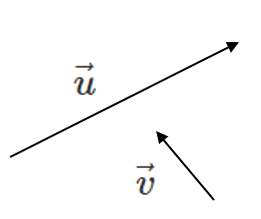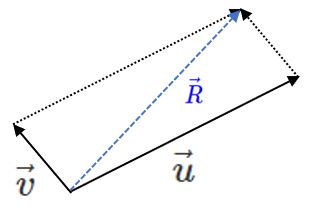## Adding Geometric Vectors Tail to Tail

Adding vectors results in a new Resultant Vector. Geometric and algebraic vectors can be added using different techniques.

Geometric vectors can be added by positioning the vectors head to tail or tail to tail. Remember, you can move a vector around in space as long as it maintains its magnitude and direction.

## Adding Geometric Vectors Tail to Tail

To add vectors tail to tail, move one vector so that it's tail starts at the tail of the previous vector. Next, make a parallelogram. The Resultant Vector starts at the tail of the vectors and ends at the opposite side of the parallelogram.

Below are the steps to add vectors $$\vec{u}$$ and $$\vec{v}$$ tail to tail. Sometimes, this method is referred to the Parallelogram Law of Vector Addition.Notice that this method is very similar to the Triangle Law of Vector Addition. By moving vector $$\vec{v}$$ over to the head of $$\vec{u}$$ makes a triangle. However, sometimes the exact direction of the vectors is unknown. Instead, you may be provided information about the angle between the vectors. That is when the Parallelogram Law of Vector Addition is most useful.

Adding geometric vectors involves solving triangles using trigonometry equations. Below is a summary:

 Right Triangles Pythagorean Theorem $$c^2 = a^2 + b^2$$ SOH CAH TOA $$sin(\theta) = \frac{opp}{hyp} = \frac{y}{r}$$ $$cos(\theta) = \frac{adj}{hyp} = \frac{x}{r}$$ $$tan(\theta) = \frac{opp}{adj} = \frac{y}{x}$$
 Other Triangles Cosine Law $$c^2 = a^2 + b^2 - 2 a b cos(\theta)$$ Sin Law $$\frac{sin(A)}{a} = \frac{sin(B)}{b} = \frac{sin(C)}{c}$$
##### Try these questions:

Frac and Michelle take their son Marco tobogganing. On the way to the slopes, Macro sits on the toboggan and Frac and Michelle each pull on a string attached to the front. Frac applies a force of 45 N away from the center of the toboggan. Michelle applies a force of 26 N away from the center of the toboggan in the other direction. The angle between the strings is $$60^\circ$$. What is the resultant force applied to Marco on the toboggan?